# Digital encoder simulation using Pspice : tutorial 15

In this tutorial I will explain you the working of a simple digital system known as a digital encoder. At the start a brief and concise introduction to encoders specifically a simplest 4×2 encoder is provided with the explanation of the output they will show. An encoder is the opposite of a decoder or a multiplexer. It is used to encode the numbers into binary digits as you will see shortly. After that the circuits is simulated using PSPICE and the result are compared with the theoretical discussion provided (which should be same). You are provided with an exercise to do it by yourself, at the end of the tutorial and in the next tutorials I will assume that you have done those exercises and I will not explain the concept regarding them.

### Introduction to digital encoder

An encoder in its simplest terms is a circuit, a device, a software program or a person that can convert information from one format or type to another. In digital world, however, encoders are named as devices which can change the binary digits from one format to another. The block diagram of a simple 4×2 encoder is shown in the figure below,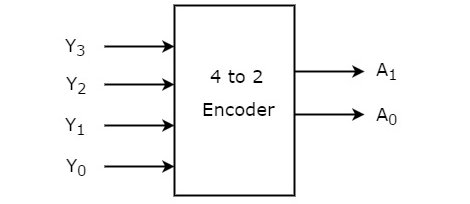Figure 1: Block diagram of encoder

This encoder will encode a four-bits binary number in two-bit binary number. The internal diagram of a simple 4×2 encoder is shown in the figure below,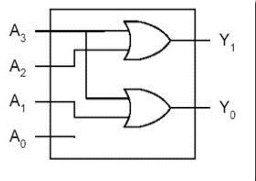Figure 2: Encoder circuit diagram

If the value of A0 is 1 then then output will represent a binary 0 and if A1 is 1 and all others are zero, then the output will be a binary 1. The truth table of a 4×2 encoder is shown in the figure below,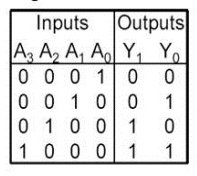Figure 3: Truth table of encoder

This implies that when A0 is 1 the output will represent 0 (00) and when A3 is 1 the output will show 3 (11) as is obvious from the above truth table.

### Digital encoder simulation using PSpice

• Encoder in its simplest form is a 4×2 encoder. By 4×2 we mean that the number of inputs is 4 (the first term of the name) and the number of outputs is 2. An encoder as we have discussed above is used to encode the digital numbers from one format to another. Lets’ know design the circuit for an encoder on PSPICE using the techniques we used previously. Write design manager in the search bar of your laptop. PSPICE design manager will open, after that click on the run schematic button to open the schematic as shown in the figure below,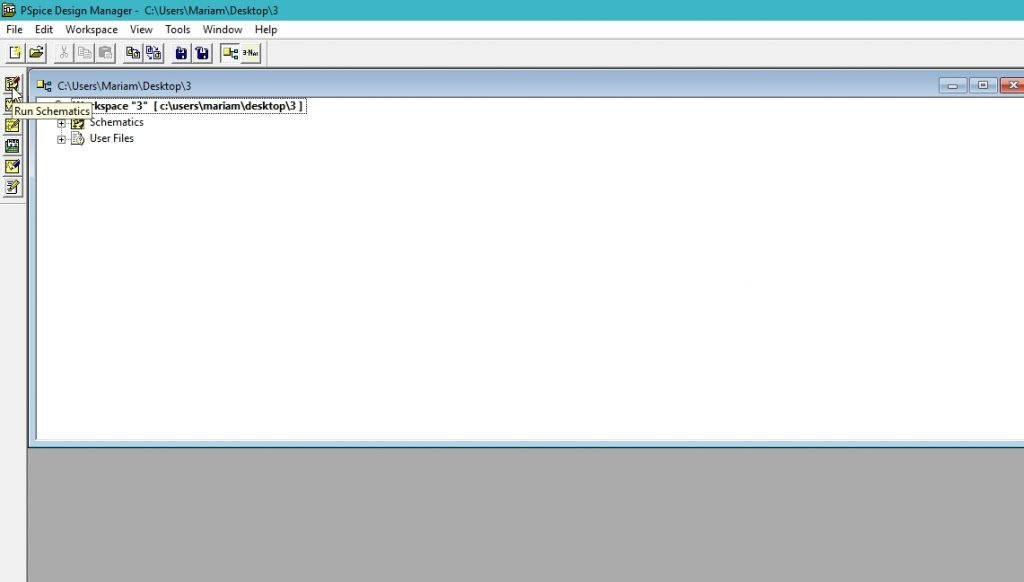Figure 4: Opening new schematic

Figure 5: Saving schematic

Figure 6: Getting new part

Figure 7: Placing OR gate

Figure 8: Placing digital source

Figure 9: Placed components

Figure 10: Drawing wire

Figure 11: Complete circuit diagram

• The digital source at the bottom of the circuit diagram is represent the input source A0. As you can see from the figure of the circuit diagram in the introduction part A0 is not connected to any component as shown in the circuit diagram above. Next step is to place the voltage marker at the input and output nodes of the circuit. A voltage marker is placed at node where we want to see examine the voltage. From the top of the schematic select voltage marker as shown in the figure below,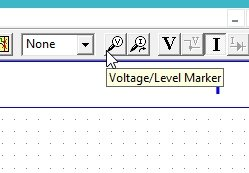Figure 12: Voltage marker

Figure 13: Voltage marker

Figure 14: Labeling a line

Figure 15: Labeled circuit diagram

Figure 16: Labeling a device

Figure 17: Labeled devices.

Figure 18: Attributes of A0

Figure 19: Attributes of A1

Figure 20: Attributes of A2

Figure 21: Attributes of A3

Figure 22: Simulation setup

Figure 23: Transient block

Figure 24: Simulation

Figure 25: Output of the encoder

If we compare the output of the encoder with the truth table they both will be same.

Exercise:

• Design a 8×3 encoder using the same techniques provided in this tutorial.